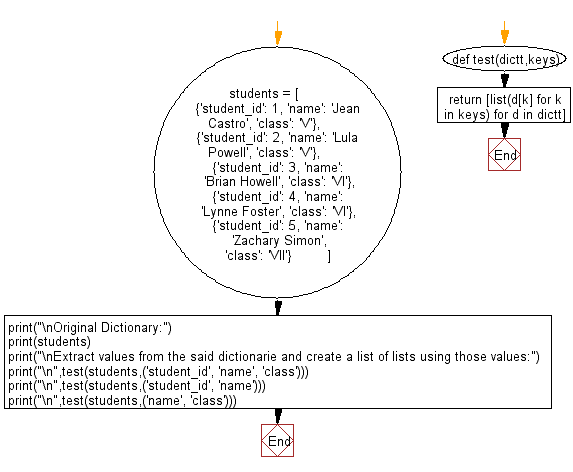﻿ Python: Extract values from a given dictionaries and create a list of lists from those values - w3resource# Python: Extract values from a given dictionaries and create a list of lists from those values

## Python dictionary: Exercise-62 with Solution

Write a Python program to extract values from a given dictionaries and create a list of lists from those values.

Sample Solution:

Python Code:

``````def test(dictt,keys):
return [list(d[k] for k in keys) for d in dictt]

students = [
{'student_id': 1, 'name': 'Jean Castro', 'class': 'V'},
{'student_id': 2, 'name': 'Lula Powell', 'class': 'V'},
{'student_id': 3, 'name': 'Brian Howell', 'class': 'VI'},
{'student_id': 4, 'name': 'Lynne Foster', 'class': 'VI'},
{'student_id': 5, 'name': 'Zachary Simon', 'class': 'VII'}
]

print("\nOriginal Dictionary:")
print(students)
print("\nExtract values from the said dictionarie and create a list of lists using those values:")
print("\n",test(students,('student_id', 'name', 'class')))
print("\n",test(students,('student_id', 'name')))
print("\n",test(students,('name', 'class')))
```
```

Sample Output:

```Original Dictionary:
[{'student_id': 1, 'name': 'Jean Castro', 'class': 'V'}, {'student_id': 2, 'name': 'Lula Powell', 'class': 'V'}, {'student_id': 3, 'name': 'Brian Howell', 'class': 'VI'}, {'student_id': 4, 'name': 'Lynne Foster', 'class': 'VI'}, {'student_id': 5, 'name': 'Zachary Simon', 'class': 'VII'}]

Extract values from the said dictionarie and create a list of lists using those values:

[[1, 'Jean Castro', 'V'], [2, 'Lula Powell', 'V'], [3, 'Brian Howell', 'VI'], [4, 'Lynne Foster', 'VI'], [5, 'Zachary Simon', 'VII']]

[[1, 'Jean Castro'], [2, 'Lula Powell'], [3, 'Brian Howell'], [4, 'Lynne Foster'], [5, 'Zachary Simon']]

[['Jean Castro', 'V'], ['Lula Powell', 'V'], ['Brian Howell', 'VI'], ['Lynne Foster', 'VI'], ['Zachary Simon', 'VII']]
```

Pictorial Presentation:Flowchart:## Visualize Python code execution:

The following tool visualize what the computer is doing step-by-step as it executes the said program:

Python Code Editor:

Have another way to solve this solution? Contribute your code (and comments) through Disqus.

What is the difficulty level of this exercise?

Test your Programming skills with w3resource's quiz.

﻿

## Python: Tips of the Day

Clamps num within the inclusive range specified by the boundary values x and y:

Example:

```def tips_clamp_num(num,x,y):
return max(min(num, max(x, y)), min(x, y))
print(tips_clamp_num(2, 4, 6))
print(tips_clamp_num(1, -1, -6))
```

Output:

```4
-1
```